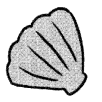# Math in Focus Grade 3 Chapter 6 Practice 6 Answer Key Multiply by 9

Go through the Math in Focus Grade 3 Workbook Answer Key Chapter 6 Practice 6 Division: Finding the Number of Items in Each Group to finish your assignments.

## Math in Focus Grade 3 Chapter 6 Practice 6 Answer Key Multiply by 9

Write two related division sentences.

Example
6 × 7 = 42
42 ÷ 6 = 7
42 ÷ 7 = 6

Question 1.
9 × 5 = 45
45 ÷ ___ = ___
45 ÷ ___ = ___
45 ÷9 = 5,
45 ÷5 = 9.

Explanation:
Given that 9 × 5 is 45,
45 ÷9 = 5 and
45 ÷5 = 9.

Question 2.
7 × 9 = 63
63 ÷ ___ = ___
63 ÷ ___ = ___
63 ÷ 7 = 9,
63 ÷ 9 = 7.

Explanation:
Given that 7 × 9 is 63,
63 ÷ 7 = 9,
63 ÷ 9 = 7.

Question 3.
8 × 6 = 48
48 ÷ ___ = ___
48 ÷ ___ = ___
48 ÷ 8 = 6,
48 ÷ 6 = 8.

Explanation:
Given that 8 × 6 is 48,
48 ÷ 8 = 6,
48 ÷ 6 = 8.

Fill in the missing numbers.

Example

6 × 7 = 42
So, 42 ÷ 6 = 7

Question 4.
7 × ___ = 49 So, 49 ÷ 7 = ____
The missing number is 7.

Explanation:
Given that the division is 49 ÷ 7 which is 7,
So 7 × 7 is 49.

Question 5.
8 × ___ = 48 So, 48 ÷ 8 = ____
The missing number is 6.

Explanation:
Given that the division is 48 ÷ 8 which is 6,
so 8 × 6 is 48.

Question 6.
9 × ___ = 45 So, 45 ÷ 9 = ____
The missing number is 5.

Explanation:
Given that the division is 45 ÷ 9 is 5,
so 9 × 5 = 45.

Solve.

Question 7.
Mrs. Brown has 9 purses with 54 coins. Each purse has the same number of coins. How many coins does each purse have?
____ ÷ ___ = ____
Each purse has __________ coins.
Each purse has 6 coins.

Explanation:
Given that Mrs. Brown has 9 purses with 54 coins and each purse has the same number of coins. So the number of coins does each purse has is 54÷9 which is 6 coins. So each purse has 6 coins.

Question 8.
Austin collects 63 seashells.
He puts them equally into 7 boxes.
How many seashells does each box contain?
____ ÷ ____ = ____Each box contains ___________ seashells.